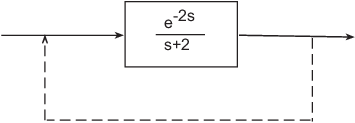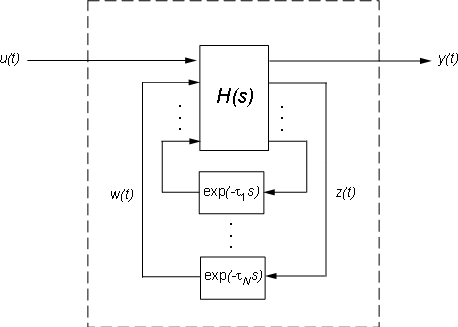Main Content

## Internal Delays

Using the `InputDelay`, `OutputDelay`, and `ioDelay` properties, you can model simple processes with transport delays. However, these properties cannot model more complex situations, such as feedback loops with delays. In addition to the `InputDelay` and `OutputDelay` properties, state-space (`ss`) models have an `InternalDelay` property. This property lets you model the interconnection of systems with input, output, or transport delays, including feedback loops with delays. You can use `InternalDelay` property to accurately model and analyze arbitrary linear systems with delays. Internal delays can arise from the following:

• Concatenating state-space models with input and output delays.

• Feeding back a delayed signal.

• Converting MIMO `tf` or `zpk` models with transport delays to state-space form.

Using internal time delays, you can do the following:

• In continuous time, generate approximate-free time and frequency simulations, because delays do not have to be replaced by a Padé approximation. In continuous time, this allows for more accurate analysis of systems with long delays.

• In discrete time, keep delays separate from other system dynamics, because delays are not replaced with poles at z = 0, which boosts efficiency of time and frequency simulations for discrete-time systems with long delays.

• Use most Control System Toolbox™ functions.

• Test advanced control strategies for delayed systems. For example, you can implement and test an accurate model of a Smith predictor. See the example Control of Processes with Long Dead Time: The Smith Predictor.

### Why Internal Delays Are Necessary

This example illustrates why input, output, and transport delays not enough to model all types of delays that can arise in dynamic systems. Consider the simple feedback loop with a 2 s. delay:The closed-loop transfer function is

`$\frac{{e}^{-2s}}{s+2+{e}^{-2s}}$`

The delay term in the numerator can be represented as an output delay. However, the delay term in the denominator cannot. In order to model the effect of the delay on the feedback loop, the `InternalDelay` property is needed to keep track of internal coupling between delays and ordinary dynamics.

Typically, you do not create state-space models with internal delays directly, by specifying the A, B, C, and D matrices together with a set of internal delays. Rather, such models arise when you interconnect models having delays. There is no limitation on how many delays are involved and how the models are connected. For an example of creating an internal delay by closing a feedback loop, see Closing Feedback Loops with Time Delays.

### Behavior of Models With Internal Delays

When you work with models having internal delays, be aware of the following behavior:

• When a model interconnection gives rise to internal delays, the software returns an `ss` model regardless of the interconnected model types. This occurs because only `ss` supports internal delays.

• The software fully supports feedback loops. You can wrap a feedback loop around any system with delays.

• When displaying the `A`, `B`, `C`, and `D` matrices, the software sets all delays to zero (creating a zero-order Padé approximation). This approximation occurs for the display only, and not for calculations using the model.

For some systems, setting delays to zero creates singular algebraic loops, which result in either improper or ill-defined, zero-delay approximations. For these systems:

• Entering `sys` returns only sizes for the matrices of a system named `sys`.

• Entering `sys.A` produces an error.

The limited display and the error do not imply a problem with the model `sys` itself.

### Inside Time Delay Models

State-space objects use generalized state-space equations to keep track of internal delays. Conceptually, such models consist of two interconnected parts:

• An ordinary state-space model H(s) with an augmented I/O set

• A bank of internal delays.The corresponding state-space equations are:

`$\begin{array}{l}\stackrel{˙}{x}=Ax\left(t\right)+{B}_{1}u\left(t\right)+{B}_{2}w\left(t\right)\\ y\left(t\right)={C}_{1}x\left(t\right)+{D}_{11}u\left(t\right)+{D}_{12}w\left(t\right)\\ z\left(t\right)={C}_{2}x\left(t\right)+{D}_{21}u\left(t\right)+{D}_{22}w\left(t\right)\\ {w}_{j}\left(t\right)=z\left(t-{\tau }_{j}\right),\text{ }j=1,...,N\end{array}$`

You need not bother with this internal representation to use the tools. If, however, you want to extract `H` or the matrices `A`, `B1`, `B2`, `...` , you can use `getDelayModel`, For the example:

```P = 5*exp(-3.4*s)/(s+1); C = 0.1 * (1 + 1/(5*s)); T = feedback(ss(P*C),1); [H,tau] = getDelayModel(T,'lft'); size(H)```

Note that `H` is a two-input, two-output model whereas `T` is SISO. The inverse operation (combining `H` and `tau` to construct `T`) is performed by `setDelayModel`.

See ,  for details.

### Functions That Support Internal Time Delays

The following commands support internal delays for both continuous- and discrete-time systems:

#### Limitations on Functions that Support Internal Time Delays

The following commands support internal delays for both continuous- and discrete-time systems and have certain limitations:

### Functions That Do Not Support Internal Time Delays

The following commands do not support internal time delays:

### References

 P. Gahinet and L.F. Shampine, "Software for Modeling and Analysis of Linear Systems with Delays," Proc. American Control Conf., Boston, 2004, pp. 5600-5605

 L.F. Shampine and P. Gahinet, Delay-differential-algebraic Equations in Control Theory, Applied Numerical Mathematics, 56 (2006), pp. 574-588

## Support

#### Learn how to automatically tune PID controller gains

Download code examples